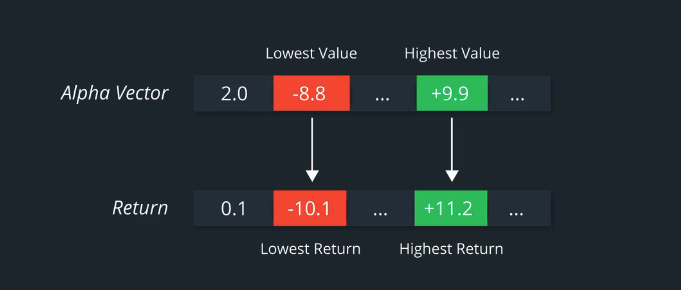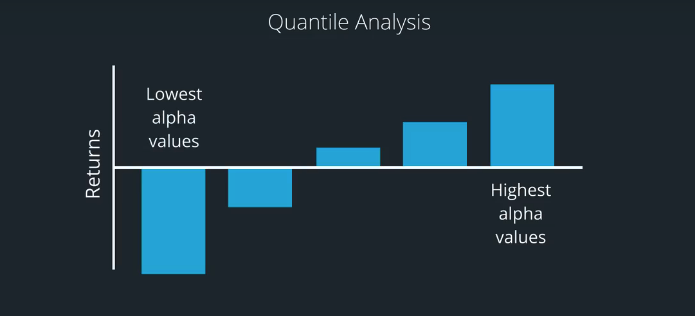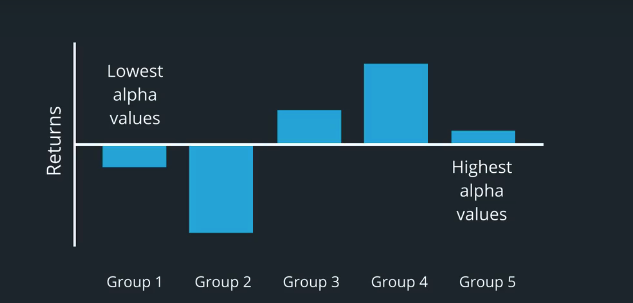# AI For Trading:Quantile Analysis (72)

## Quantile Analysis

We saw earlier that the rank IC can tell us overall how well an Alpha vector's predictions align with the subsequent stock returns for the next period.

You may be wondering if it's possible to drill down deeper to look at the Alpha values assigned each stock to see which subset of stocks actually contribute most or least to the factor return of the portfolio.

Ideally, if our Alpha model and hypothesis were accurate, the stock with the highest Alpha value for that day would also have the highest positive return the next day.

Similarly, the stock with the lowest Alpha value for that day would also have the lowest return in the next day, which would be good for our theoretical portfolio since we would be shorting that stock.Remember, that we are working with perhaps hundreds or even thousands of stocks in our universe. So, a good middle ground is to divide our Alpha vector into quantiles and analyze their returns and volatility within those quantiles.An ideal Alpha model would be one where the group of stocks containing the highest Alpha values for that day would also have the highest average return, and possibly, the highest risk adjusted to return.

Similarly, the group of stocks containing the lowest Alpha values would ideally have the lowest returns. For example, if we have 25 stocks in our universe and wanted divide them up each into five groups of equal size, we would be using five quantiles also called quintiles.

For each day, we'll sort the stocks by their Alpha value.The five highest values go in the fifth group, which are above the fourth quintile.

The stocks with the lowest five values go in the first group, which are below the first quintile.Similarly, we fill groups 2, 3,4, with five stocks each based on their Alpha value.

We can keep track of the individual returns within each of the five groups over a time window such as one,three, or five years.

Then we can calculate the mean return within each group as well as the standard deviation. We can call what we just did quantile analysis or quantile performance.

Since we chose five quantiles, we can also call this quintile performance.## mean returns by quantile quiz

### mean_returns_by_quantile

Here is the documentation for alphalens mean_return_by_quantile

Here is the source code
Review these to answer the quiz below.

For the mean_returns_by_quantile function, by default, are the quantiles the same across all time periods of the data? or are quantiles calculated for each time period?
A: same across all time periods
B: calculated for each time peroid

## Install packages

import sys
!{sys.executable} -m pip install -r requirements.txt
Collecting alphalens==0.3.2 (from -r requirements.txt (line 1))
Using cached https://files.pythonhosted.org/packages/a5/dc/2f9cd107d0d4cf6223d37d81ddfbbdbf0d703d03669b83810fa6b97f32e5/alphalens-0.3.2.tar.gz
Collecting colour==0.1.5 (from -r requirements.txt (line 2))
Using cached https://files.pythonhosted.org/packages/74/46/e81907704ab203206769dee1385dc77e1407576ff8f50a0681d0a6b541be/colour-0.1.5-py2.py3-none-any.whl
Collecting cvxpy==1.0.3 (from -r requirements.txt (line 3))
Using cached https://files.pythonhosted.org/packages/a1/59/2613468ffbbe3a818934d06b81b9f4877fe054afbf4f99d2f43f398a0b34/cvxpy-1.0.3.tar.gz
Requirement already satisfied: cycler==0.10.0 in /Users/kaiyiwang/anaconda3/lib/python3.7/site-packages (from -r requirements.txt (line 4)) (0.10.0)
Requirement already satisfied: numpy==1.14.5 in /Users/kaiyiwang/anaconda3/lib/python3.7/site-packages (from -r requirements.txt (line 5)) (1.14.5)
Collecting pandas==0.18.1 (from -r requirements.txt (line 6))
Using cached https://files.pythonhosted.org/packages/11/09/e66eb844daba8680ddff26335d5b4fead77f60f957678243549a8dd4830d/pandas-0.18.1.tar.gz
import cvxpy as cvx
import numpy as np
import pandas as pd
import time
import os
import quiz_helper
import matplotlib.pyplot as plt
%matplotlib inline
plt.style.use('ggplot')
plt.rcParams['figure.figsize'] = (14, 8)

### data bundle

import os
import quiz_helper
from zipline.data import bundles
os.environ['ZIPLINE_ROOT'] = os.path.join(os.getcwd(), '..', '..','data','module_4_quizzes_eod')
ingest_func = bundles.csvdir.csvdir_equities(['daily'], quiz_helper.EOD_BUNDLE_NAME)
bundles.register(quiz_helper.EOD_BUNDLE_NAME, ingest_func)
print('Data Registered')

### Build pipeline engine

from zipline.pipeline import Pipeline
from zipline.pipeline.factors import AverageDollarVolume
from zipline.utils.calendars import get_calendar

universe = AverageDollarVolume(window_length=120).top(500)
engine = quiz_helper.build_pipeline_engine(bundle_data, trading_calendar)

### View Data

With the pipeline engine built, let's get the stocks at the end of the period in the universe we're using. We'll use these tickers to generate the returns data for the our risk model.

universe_end_date = pd.Timestamp('2016-01-05', tz='UTC')

universe_tickers = engine\
.run_pipeline(
Pipeline(screen=universe),
universe_end_date,
universe_end_date)\
.index.get_level_values(1)\
.values.tolist()

universe_tickers

Get Returns data

from zipline.data.data_portal import DataPortal

data_portal = DataPortal(
bundle_data.asset_finder,
adjustment_reader=bundle_data.adjustment_reader)

## Get pricing data helper function

def get_pricing(data_portal, trading_calendar, assets, start_date, end_date, field='close'):
end_dt = pd.Timestamp(end_date.strftime('%Y-%m-%d'), tz='UTC', offset='C')
start_dt = pd.Timestamp(start_date.strftime('%Y-%m-%d'), tz='UTC', offset='C')

return data_portal.get_history_window(
assets=assets,
end_dt=end_dt,
bar_count=end_loc - start_loc,
frequency='1d',
field=field,
data_frequency='daily')

## get pricing data into a dataframe

returns_df = \
get_pricing(
data_portal,
universe_tickers,
universe_end_date - pd.DateOffset(years=5),
universe_end_date)\
.pct_change()[1:].fillna(0) #convert prices into returns

returns_df

## Sector data helper function

We'll create an object for you, which defines a sector for each stock. The sectors are represented by integers. We inherit from the Classifier class. Documentation for Classifier, and the source code for Classifier

from zipline.pipeline.classifiers import Classifier
from zipline.utils.numpy_utils import int64_dtype
class Sector(Classifier):
dtype = int64_dtype
window_length = 0
inputs = ()
missing_value = -1

def __init__(self):

def _compute(self, arrays, dates, assets, mask):
return np.where(
self.data[assets],
self.missing_value,
)
sector = Sector()

## We'll use 2 years of data to calculate the factor

Note: Going back 2 years falls on a day when the market is closed. Pipeline package doesn't handle start or end dates that don't fall on days when the market is open. To fix this, we went back 2 extra days to fall on the next day when the market is open.

factor_start_date = universe_end_date - pd.DateOffset(years=2, days=2)
factor_start_date

## Create smoothed momentum factor

from zipline.pipeline.factors import Returns
from zipline.pipeline.factors import SimpleMovingAverage

# create a pipeline called p
p = Pipeline(screen=universe)
# create a factor of one year returns, deman by sector, then rank
factor = (
demean(groupby=Sector()). #we use the custom Sector class that we reviewed earlier
rank().
zscore()
)

# Use this factor as input into SimpleMovingAverage, with a window length of 5
# Also rank and zscore (don't need to de-mean by sector, s)
factor_smoothed = (
SimpleMovingAverage(inputs=[factor], window_length=5).
rank().
zscore()
)

# add the unsmoothed factor to the pipeline
# add the smoothed factor to the pipeline too
p.add(factor_smoothed, 'Smoothed_Momentum_Factor')

## visualize the pipeline

Note that if the image is difficult to read in the notebook, right-click and view the image in a separate tab.

p.show_graph(format='png')

## run pipeline and view the factor data

df = engine.run_pipeline(p, factor_start_date, universe_end_date)
df.head()

## Evaluate Factors

We'll go over some tools that we can use to evaluate alpha factors. To do so, we'll use the alphalens library

## Import alphalens

import alphalens as al

## Get price data

Note, we already got the price data and converted it to returns, which we used to calculate a factor. We'll retrieve the price data again, but won't convert these to returns. This is because we'll use alphalens functions that take their input as prices and not returns.

## Define the list of assets

Just to make sure we get the prices for the stocks that have factor values, we'll get the list of assets, which may be a subset of the original universe

# get list of stocks in our portfolio (tickers that identify each stock)
assets = df.index.levels.values.tolist()
print(f"stock universe number of stocks {len(universe_tickers)}, and number of stocks for which we have factor values {len(assets)}")
factor_start_date
pricing = get_pricing(
data_portal,
assets, #notice that we used assets instead of universe_tickers; in this example, they're the same
factor_start_date, # notice we're using the same start and end dates for when we calculated the factor
universe_end_date)
factor_names = df.columns
print(f"The factor names are {factor_names}")

# Use a dictionary to store each dataframe, one for each factor and its associated forward returns
factor_data = {}
for factor_name in factor_names:
print("Formatting factor data for: " + factor_name)
# Get clean factor and forward returns for each factor
# Choose single period returns (daily returns)
factor_data[factor_name] = al.utils.get_clean_factor_and_forward_returns(
factor=df[factor_name],
prices=pricing,
periods=)

## quantile analysis

alphalens.performance.mean_return_by_quantile(factor_data,
...
demeaned=True,
...)
• factor_data: A MultiIndex DataFrame indexed by date (level 0) and asset (level 1), containing the values for a single alpha factor, forward returns for each period, the factor quantile/bin that factor value belongs to, and (optionally) the group the asset belongs to
• demeaned: this is True by default. This makes a call to alphalens.utils.demean_forward_returns, which takes each factor return and subtracts the mean of all the factor returns in our universe of stocks.
• returns:mean_ret : pd.DataFrame: Mean period wise returns by specified factor quantile.
• Note that it returns a second variable, standard error of the returns. We will focus on the mean return.

## Quiz 1

Look at the error message when trying to call the mean_return_by_quantile function, passing in the factor data. What data type is required?

factor_names = df.columns
ls_fra = []

for i, factor_name in enumerate(factor_names):
print("Calculating the FRA for: " + factor_name)

#TODO: look at the error generated from this line of code
quantile_return, quantile_stderr = al.performance.mean_return_by_quantile(factor_data[factor_name])
quantile_return.columns = [factor_name]
qr_factor_returns.append(quantile_return)

df_ls_fra = pd.concat(ls_fra, axis=1)

An integer is required

## Convert datetime to integer

To pass in factor data that the factor_rank_autocorrelation function can use, we'll convert the datetime into an integer using unix

unixt_factor_data = {}
for factor_name in factor_names:
unixt_index_data = [(x.timestamp(), y) for x, y in factor_data[factor_name].index.values]
unixt_factor_data[factor_name] = factor_data[factor_name].set_index(pd.MultiIndex.from_tuples(unixt_index_data, names=['date', 'asset']))

## Quiz 2:

Calculate the quantile returns.

Use the data for which the datetime index was converted to integer

factor_names = df.columns
ls_qr = []

for i, factor_name in enumerate(factor_names):
print("Calculating the FRA for: " + factor_name)

#TODO: calculate quantile returns and standard errors
# store the quantile returns
quantile_return, quantile_stderr = al.performance.mean_return_by_quantile(unixt_factor_data[factor_name])
quantile_return.columns = [factor_name]
ls_qr.append(quantile_return)
df_ls_qr = pd.concat(ls_qr, axis=1)

## View the outputted FRA

df_ls_qr.plot.bar(
subplots=True,
sharey=True,
layout=(4,2),
figsize=(14, 14),
legend=False,
title='Alphas Comparison: Per Day per Quantile'
);

## Quiz 3

How would you compare the quantile returns of the unsmoothed and smoothed factors? Which one would you prefer based on just the quantile returns?

The smoothed factor appears to have a more balanced distribution (more of the predictive power is distributed across more of the stocks in the portfolio). The unsmoothed appears to generate most of its returns from the tails (the highest and lowes quantiles). We'd prefer the smoothed factor because it relies on more of the stocks in the portfolio for the returns, instead of just the tail ends of the distribution of stocks.

## Quiz 4 basis points

Notice how the y-axis has pretty small numbers for the percentages. We normally use basis points as the unit of measurement. To convert from decimal (e.g. 0.01 is one percent) to basis points, multiply by $10^4$.

# TODO: convert values to basis points
df_ls_qr_bp = 10000 * df_ls_qr
df_ls_qr_bp

## re-plot using basis point scaling

df_ls_qr_bp.plot.bar(
subplots=True,
sharey=True,
layout=(4,2),
figsize=(14, 14),
legend=False,
title='Alphas Comparison: Per Day per Quantile (basis points)'
);